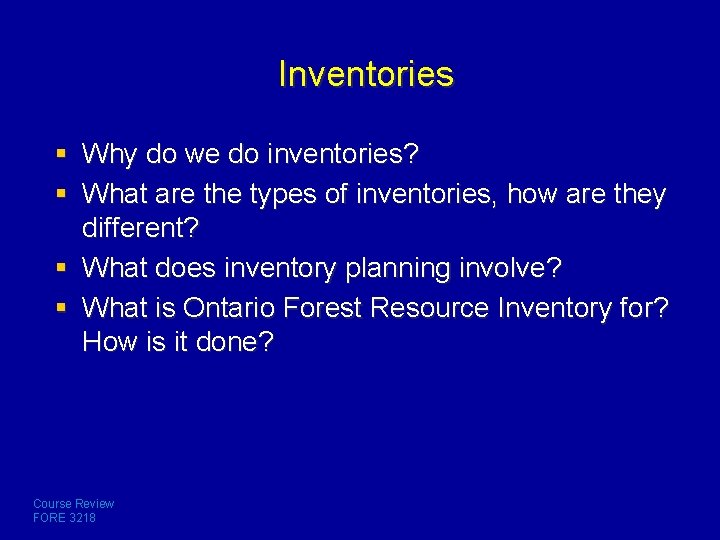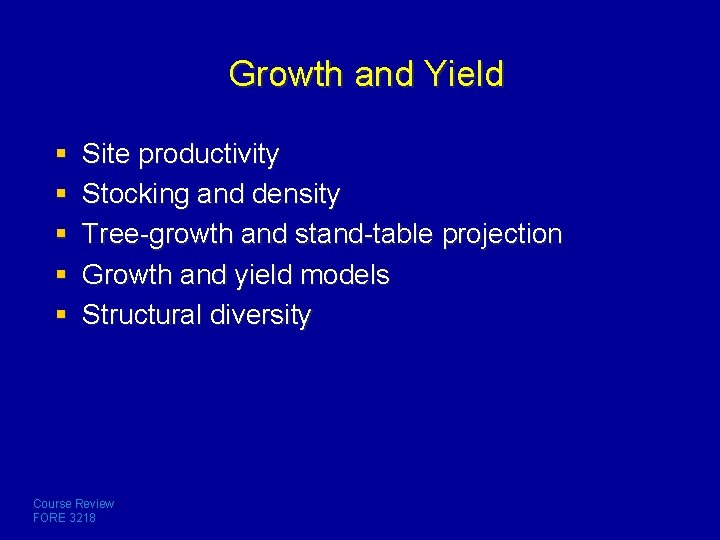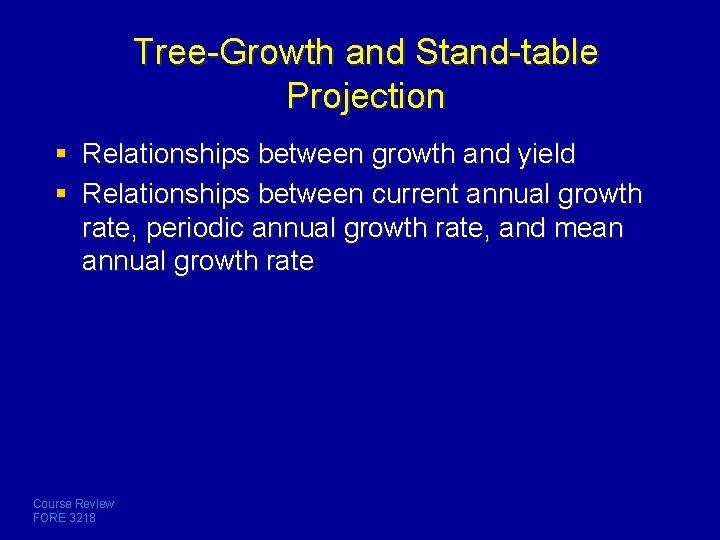# Course Review Sampling Inventories Growth and yield Course

• Slides: 18Course Review § Sampling § Inventories § Growth and yield Course Review FORE 3218Course Introduction § What are forest measurements? § Why measure forest resources? § What are the types and scales of measurements? Can you identify them? Course Review FORE 3218Statistical Methods § § § Bias, accuracy, and precision Calculating probabilities Population vs sample Parameter and variable Meaning and types of frequency distribution Measures of average population variables; Know to calculate them § Measures of dispersion and their calculations § Meaning and calculation of confidence limits Course Review FORE 3218Elementary sampling § Why sampling? § Definitions of sample, sampling unit, and sampling frame § Advantages and disadvantages of selective, simple random, and systematic sampling methods § Procedures of a simple random sampling § How to estimate population parameters from a simple random sample? § Sampling intensity? How to determine and what are the factors influencing its determination? § Why systematic sampling? Course Review FORE 3218Stratified random sampling § How is it similar to and different from simple random sampling? § Why, when, and how do we use it? § How to calculate the mean, measures of dispersion, and confidence limit from a stratified random sample? Course Review FORE 3218Regression and ratio estimation § What is regression? § What are the meaning of b 0 and b 1 in a linear regression? § How do you measure the fit of a regression? § Purpose of regression estimation as a sampling method? When can we use it? § How regression estimation is compared with simple random sampling? § Meaning of ratio estimation? Course Review FORE 3218Sampling Elementary sampling methods: – selective, simple random, and systematic § Stratified random sampling § Regression and ratio estimation § Double sampling, cluster, and sampling for discrete variables Course Review FORE 3218 § What are their characteristics? § Can you calculate simple statistics? – mean and dispersion – sample size determination § Can you give some examples?Inventories § Why do we do inventories? § What are the types of inventories, how are they different? § What does inventory planning involve? § What is Ontario Forest Resource Inventory for? How is it done? Course Review FORE 3218Inventory with fixed-area plots or strips § Line-plot system cruising – How is done? – Can you determine line distance or space between plots with known sampling density? § Permanent sample plots – – – What is it for? How is normally done? Why do not we establish more PSPs? Course Review FORE 3218Inventory with point samples § What are factors affecting the probability of tallying a tree? § Basal area factor (BAF) – What is it? – What affects the BAF of a prism? – How could you determine the BAF of a prism? § Plot radius factor – What does it mean? – How can it be calculated? Course Review FORE 3218 § Why each tallied tree represents the same basal area on a per-unitarea basis? § Calculations for point samples – Determination of stand basal area, stand density, and stand volume?Growth and Yield § § § Site productivity Stocking and density Tree-growth and stand-table projection Growth and yield models Structural diversity Course Review FORE 3218Site Productivity § What is site quality? § How you may determine site quality in different situations? Course Review FORE 3218Stand Density and Stocking § § Definition of stand density and stocking Types of measurements How are they measured at the stand level? How is point density measured? Course Review FORE 3218Tree-Growth and Stand-table Projection § Relationships between growth and yield § Relationships between current annual growth rate, periodic annual growth rate, and mean annual growth rate Course Review FORE 3218Growth and Yield Models § § Definition of growth and yield Relationships between growth and yield What are the measures of growth and yield? What are the types of growth and yield models and their attributes? § How many models have you heard? Course Review FORE 3218Stand Structural Diversity § § § Definition How it can be classified? How can it be quantified? Course Review FORE 3218What are measured in the final exam? § How well you understood lectures, including classroom examples § Whether you could accomplish your lab assignments independently § Whether you have done your assignments (reading and excises) Course Review FORE 3218Good luck! Course Review FORE 3218